# Using the Function Data Type

`function` is a StreamBase data type and a reserved keyword. Use a constructor for the function data type to create a custom expression language function whose components are built-in functions, math operators, and even other functions. A StreamBase expression you use often can be expressed as a function for efficient reuse. You can pass fields with the function data type to other components in a stream.

## Defining Custom Functions

Define an expression language function with the following function literal syntax:

`function `label` (arg1 T, arg2 T, ...) `-> returnType` { `body` }`

Parentheses enclose the list of arguments for this function. Braces enclose the body of the function definition.

The `label` for the function is its optional internal name. You can use the optional `label` to document the purpose of the function. The `label` is only required if the function being defined calls itself recursively. This is the case in the formula to emit the `nth` member of the Fibonacci series shown here:

`function fib (n int) -> int {if n <= 1 then n else fib(n-1) + fib(n-2)}`

When used as a recursion name, the `label` has scope only within that function.

In the usual case, a defined function's return type is the same for all output values. Thus, the usual syntax is without the optional `returnType` argument:

`function `label` (arg1 T, arg2 T, ...) { `body` }`

If you do specify a return data type prefixed by the two characters "`->`", that data type must be compatible with the function's output. If you leave out the `returnType` argument, StreamBase determines the return type based on what the expression body does to its inputs. For further details, see Data Type Coercion and Conversion.

The function body contains a valid StreamBase expression, such as the following, which accepts a double value, the temperature in Celsius degrees, and returns the same temperature on the Fahrenheit scale:

`function (c double) { round( c * 9/5 + 32 , 2) }`

The names you assign to arguments do not need to be used in the function body, but if so, they must be the exact same names. Do not try to assign different names for the same value as an argument and in the function body. (However, you can @define new names in the function body as described below.)

You can have multiple expressions inside a function body, with each line terminated by a semicolon. A semicolon after the last statement is allowed but ignored. The return value of the function is that of the last expression on the block. For example:

```function (a int, b int) {
/* First define the sq() and
sumsq() internal functions */
@define (function (y int) { y * y } as sq);
@define (function (c int, d int) { sq(c) + sq(d) } as sumsq);
sumsq(a, b) --Final semicolon is allowed but not required
} ```

As shown above, you can use either C-style comments on one or more lines or legacy StreamBase double-hyphen comments which are valid to the end of their line. These are the standard comment styles for the StreamBase expression language.

## The Expression Evaluator and Custom Functions

The Evaluate StreamBase Expression dialog is especially useful when defining functions. Open the dialog with Help>Evaluate StreamBase Expression to experiment with your function definition syntax until the dialog confirms it is valid. When the Evaluator approves, you can copy your function and paste it into the context where you will define it.

The Evaluator accepts the full multiline syntax for function definitions, and recognizes comments. As an example, copy the entire last example from the previous section into the Evaluator and click . When a function definition is valid, the Evaluator shows you a Result line, and it restates the entire expression on a single line, adding return values and other assumed values.

You can provide arguments to expressions in the Evaluator to see your function expression in action, without having to start and run an EventFlow fragment. Just add the number and type of values that the expression expects, enclosed by parentheses and placed after the close-brace of the expression definition. When you click , this time the Evaluator runs your expression with the provided values, and shows the calculated result.

For the last example of the previous section copied and pasted into the Evaluator, add `(2,3)` to the end of the function definition. The Evaluator returns 13. Provide any two other integers to confirm that the function works as expected.

For functions that accept function arguments, you can provide a function literal as an argument within parentheses.

## Internal JSON Format

StreamBase maintains function definitions internally as JSON strings. You may see the JSON format for function definitions:

• In the Input Streams view, after you've entered a function literal for a function field in the Manual Input view.

• In the Output Streams view, as the return value for a function declaration.

• In the Manual Input view, after you've entered a function literal for a function field and then returned to that Manual Input view.

• In error messages.

• In the Expression Evaluator, described above.

If your application requires it, you can define functions in JSON format. For assistance, see the tojson(), parsejson(), parsejson_loose(), and jsonpath() expression language functions.

For example, the function literal for the Celsius to Fahrenheit function shown earlier could be defined with the following JSON string.

`{"function_definition":"function (c double) { round( c * 9/5 + 32 , 2) }"}`

## Where to Define Functions

You can define functions in the following places in StreamBase Studio:

You can define and use custom expression language functions in the Expression column of the Additional Expressions grid of the Map, Query, and Iterate operators. You can do this in two ways:

Inline as part of a larger expression

You can use a function literal as part of a larger expression, possibly defined on more than one line. You can also use the `@define-as` construction described below to define an inline function and give it a name. In general, functions defined within a larger expression can be used only within that expression.

As the entire expression for a field

When the entire expression for a field is a function literal, the data type of that field is `function`. That field can then be used downstream as the name of a defined function that takes arguments and returns a result. This construction is illustrated in the Function Data Type Sample included with Studio, in the `DefineFn` and `UsePassedFn` Map operators: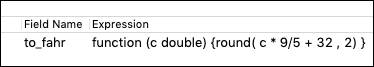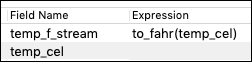EventFlow Editor, Definitions Tab

You can define a function in the Definitions tab of the EventFlow Editor for a module, providing a Name for the function and specifying the function literal in the Expression field. You can then call this function by that Name anywhere in this module or its dependent modules.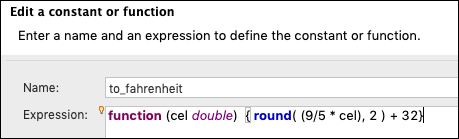Interface Editor, Definitions Tab

You can define a function in the Definitions tab of a StreamBase Interface file, then import that interface into several modules of a complex EventFlow application. The same function can then be used in any of those modules, but managed and edited in a single location.

The Studio Function Data Type Sample provides a module with these definitions that you can load, run, and modify.

## Names for Functions

By what name do you invoke a defined function to run it? That depends on where you defined it:

• Remember that a function's label in its function literal definition can never be used outside of that definition to invoke the function. When used in the definition of a recursive function, the label is used only within that function definition to invoke the function.

• If you use the @define-AS syntax described below, you then use the name assigned with AS to invoke the function. This name is only valid from the @define to the closing brace of the containing definition.

• If you define a constant as a function literal in the Definitions tab of an EventFlow or Interface Editor, then you can use that constant name to invoke the function wherever that module or interface has scope.

• If you assign a field the value of a function literal, you can then use the name of that field downstream in the same module to invoke that function. (See As the entire expression for a field in the previous section and Passing a Function on an Input Stream below.)

Remember that `function` is a first-class data type in StreamBase. Therefore, anything you can do with a value of any data type you can do with `function`. You can send it downstream as the value of a tuple field. You can enqueue it and dequeue it. You can send it across local and remote StreamBase container connections. This is why we do not describe functions themselves as having scope. What the `function` is a value of (a constant, a field, a dynamic variable) can be said to have scope, but the instance of type `function` itself can flow with any tuple that contains it.

## Arguments of Different Data Types

You can use expression language functions within and as arguments to other functions, as long as they accept and emit compatible data types.

You can call most built-in expression language functions as needed in your function body. The following shows Binet's alternative, non-recursive formula to calculate the `nth` member of the Fibonacci series:

`function (n int) {round((1/sqrt(5))*(pow((1+sqrt(5))/2,n)-pow((1-sqrt(5))/2,n)))}`

For example, in a module with the constant `fib` defined with this function literal, you can define a different function to compute the difference between a specified Fibonacci number and its immediate predecessor:

`function fibdiff (n int) {fib(n)-fib(n-1)}`

You can use the defined function as an argument for a built-in function:

`sqrt(fib(n))`

For simple data types, declaring the type of arguments or returns is straightforward: just specify the name of the type.

`function to_fahrenheit(cels double) -> double { (9/5 * cels) + 32 }`

To specify a tuple as an argument, specify its schema in parenthesized name type pairs, separated by commas. (This is the format for defining a schema in the legacy StreamSQL language.) Do not use the AS keyword that would be used with the tuple() constructor. For example:

`function foo (tpl (a int, b string)) { tpl.a }`

You can use the name of a named schema as an argument of type tuple:

`function foo (localname `namedsch`m, n int) {localname.`fieldname` + n}`

To specify a list as an argument, use the keyword `list` followed by the list's type in parentheses:

`function foo (myl list (int)) { myl + myl }`

The following example shows a list of tuples, where each tuple has the schema `a int, b string`:

`function foo (myl list ((a int, b string))) { unzip(myl) }`

The following argument is a tuple whose schema is a list of int plus an int:

`function foo (lint (a list(int), b int)) { lint.a[lint.b] }`

In the following, foo()'s argument is a function, one that takes an int and returns a double. The inner function `innerf` has no body declaration; therefore this argument is expected to be substituted at runtime with the name of a function defined elsewhere that takes an int and returns a double.

`function foo (innerf (a int)->double) { double() }`

For example, if the Definitions tab defines a constant whose name is `doublet` and whose value is the line above, and also defines a function named `convert()` that takes an int and returns a double, then `doublet()` could be run as:

`doublet(convert())`

Notice that the difference between specifying a tuple argument and a function argument is that the latter has its return type explicitly defined:

• `arg (a int)` defines a tuple argument.

• `arg (a int)->int` defines a function argument.

You can chain functions together in a way that can challenge your problem-solving skills. For example:

`function noway (arg (a int)->(b double)->(c string)->int)->string {"do-work"}`

This very unlikely definition of noway():

• Takes a single argument, a function ...

• That takes an int argument and returns a function ...

• That takes a double argument and returns a function ...

• That takes a string argument and returns an int.

• Meanwhile, noway() itself returns a value whose type is determined by the calculations in the body, in this case, a string.

In this section, `arg` is used only an example of an argument name, and is not a keyword in any way.

## Annotations @cacheable and @define

There are two annotations associated with function definitions, @cacheable and @define.

@cacheable

You can annotate a function as @cacheable to cache the result of an invocation of a function so that it is not evaluated again when called with the same arguments. For example:

`function @cacheable addem(a int, b int) { a + b }`

If you later call `addem(1, 2) * addem(1, 2)`, the value of `addem` is evaluated once and cached, instead of being evaluated twice.

@define

You can define and name local variables with `@define(name as expr)`. The scope of these variables is from the @define to the closing brace of the containing function. Duplicate variable definitions or variables with names identical to arguments are not allowed. For example:

```function combine (a int, b int)
{
@define(a + b AS sum, a * b AS prod);
a + b + sum + prod; --final semicolon is allowed but ignored
}```

You can @define inner functions within the definition of an outer function. In the following, the outer function is not labeled:

```function (a int, b int)
{
@define (function (y int) { y * y } as sq);
@define (function (c int, d int) { sq(c) + sq(d) } as sumsq);
sumsq(a, b)
}```

The @define construction can be used to override variables. However, notice that free variables are bound lexically. This means that if you create a function referring to a free variable `a`, the value of `a` is bound when the function is created, not when it is called. For example:

```function roundup(a double)
{
@define(function() {round(a, 2)} as bar);
@define(a+1 as a);
list( round(a, 2), bar());
}```

The `roundup()` function takes a double and returns a list of doubles. When given the input value 49.9876, it returns [50.99, 49.99]. This shows that the value of `a` in the inner function was bound to the incoming value when the inner function was created, not when it is called as `bar()` in the last line.

## Functions That Require Function Arguments

The following simple functions in the expression language, sometimes called higher order functions, allow or require passing in a function as one of their arguments to manipulate single or multiple lists.

• `filterlist()`  Removes elements of a list that fail a test provided by the input function.

• `foldleft()`  Applies an accumulator function forward over a set of lists of the same length and compatible types.

• `foldright()`  Applies an accumulator function backwards over a set of lists of the same length and compatible types.

• `maplist()`  Applies a function to corresponding elements of multiple lists and collates the results as a single list.

• `mergelist()`  Merges a list of sorted lists to produce a sorted output list. The input comparison function is used to compare elements of the lists.

In addition, `sort()` has a syntax that accepts a custom comparison function to sort a single list.

In the following example, the `foldleft()` function passes in a function that adds a list of ints to the initial value 10:

`foldleft(function addVals(a int, b int) { a+b }, 10, list(1, 2, 3, 4, 5, 6))`

This returns 31.

Remember that the label `addVals` is optional, but can add some clarity. The same invocation of `foldleft()` works without the label:

`foldleft(function (a int, b int) { a+b }, 10, list(1, 2, 3, 4, 5, 6))`

The argument of type `function` can be a built-in function, a field with data type `function`, a constant whose value is a function from the Definitions tab, or a function literal. Function literals start with the `function` keyword and use the syntax shown in the first section above. You can pass custom Java functions to these functions using function aliases that you declare in a configuration file.

Input functions to these higher order functions must obey certain rules:

• Their arguments must be compatible with the data type of their input lists.

• The number of calling arguments must equal the number of input lists (+1 for `foldleft()` and `foldright()`).

• The `filterlist()` input function must return true or false for each list element.

• Comparison functions must return integer values less than zero if the first argument is smaller than the second; greater than zero if the first argument is greater than the second; one or zero if the arguments are equal.

• Accumulator functions can be created to return tuples or lists of a specified data type.

## Using the compile() Function

The expression language's `compile()` function takes a string that defines an expression and a tuple that defines that expression's arguments and emits an executable function at runtime. The return type of `compile()` is the function data type, which can be passed downstream to other components as described on this page.

The first argument of `compile()` is equivalent to the `{body}` of a function literal. Thus, you can use `compile()` like the following example:

`compile( "(fahr_temp - 32) * 1.8", tuple(double() as fahr_temp), double() )`

Read more about `compile()` in its section of the Expression Language Functions page.

## Passing a Function on an Input Stream

Functions are traditionally used anywhere in the middle of an EventFlow module, between the Input Stream and Output Stream (or adapters). However, it is possible to configure an Input Stream having a field with the function data type, and pass a function to it along with other input fields.

Follow the steps of this extended example to configure one field of an input stream with a name and the function data type:

1. Let's say we want to send in a population count for a city, plus an estimated undercount percentage, and a formula to combine the two into a more accurate population count. Of course, you can apply the formula in a Map operator and/or by means of a function defined in the Definitions tab. But defining the formula as an Input Stream field allows you to change the formula at runtime in a long-running application, or to apply different formulas for different cities.

The following shows one long, one double, and one function field for an Input Stream: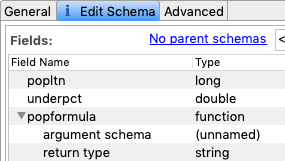2. Fill in the list of arguments for the function in much the same way as adding sub-fields to a tuple field. That is, select argument schema under `popformula` and click the button.3. Give a name and type to the first argument sub-field. Add a second argument and its type. Finally, specify the type of the return value.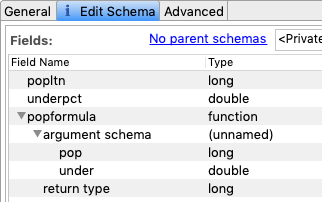4. Next, set up a Map operator to use these incoming values. Notice that the field name, `popformula`, becomes the invokable name of the function being passed from the stream. (This is a variation on the rule in the fourth bullet of Names for Functions above.)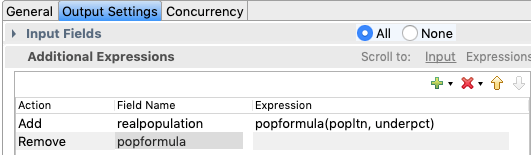5. When you run an EventFlow module with the Input Stream and Map operator described above, the Manual Input view shows three input fields.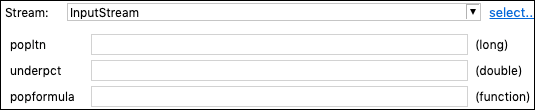6. Fill in these fields with a city's `population`, an `undercount` percentage, and the entire function literal for a function that takes the data types defined in the Input Stream's schema, a long and a double:

`function (pop long, under double) {round(pop + (pop * under))}`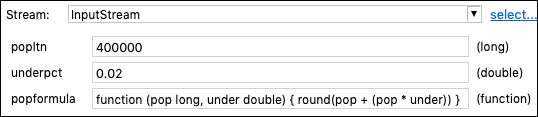7. Click . Now, look in the Output Streams view to see a tuple returned with the following values: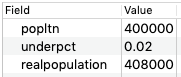### Notes

1. Open the Input Streams view after sending the above tuple. Notice that Studio has converted the function literal you typed in the Manual Input view to JSON format.

2. If you enter an impure function such as `random()` in your function literal, the result is a security error, described in the next section.

## Restrictions on Functions

1. You can define only simple expression language functions, not aggregate functions. The definition of a function cannot contain built-in aggregate functions, and the AS name in a @define-AS construction cannot use the name of an aggregate function.

2. Expression language functions from the Runtime and System categories are not expected to return useful values, or any values at all, if called in a function body.

3. A pure function is one whose output is constant or is entirely determined by its input arguments, while an impure function's output can vary every time it is run. In general, best practice is to avoid using impure functions such as `random()`, `securerandom()`, `eval()`, `calljava()`, and `timestamp()` in function definitions.

You can sometimes use impure functions in function definitions in internal operators such as Map as long as you are aware of the consequences. For example, a function data type field containing a function defined with the `random()` function in its body returns random results. If that is your intention, then your function works as intended.

However, you cannot use an impure function in a function defined in an Input Stream, or in any other external input to an EventFlow module. This is a security precaution that prevents a trespasser from entering a function on an input stream whose purpose is to destabilize the EventFlow module or its host system.

StreamBase fails to successfully compile a function definition on an Input Stream if the definition contains an impure function. The runtime error message includes wording like the following:

```... current security options prevent calling any operation that is not purely
functional from enqueued or compiled functions.```

If you encounter this error and are certain the conditions are safe for your environment, you can override the security settings, and force StreamBase to accept impure functions, by setting the system property `streambase.appgen.allow-all-function-input` to `true`.